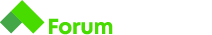# Min, max,sum, average problem

Здравейте, не мога да си открия грешката в следния код:
Някой има ли идеи?

let numbers=+gets();
let sum = 0;
let arr = [];
let min=;
let max=;
for (let i = 0; i < numbers; i++) {
arr[i] = +gets();
}
for(let i=0;i<arr.length;i++){
if(arr[i]<min){
min=arr[i];
}
}
print(min.toFixed(2));
for(let i=0;i<arr.length;i++){
if(arr[i]>max){
max=arr[i];
}
}
print(max.toFixed(2));
for(let i=0;i<arr.length;i++){
sum+=arr[i];
}
print(sum.toFixed(2));

``````let avg = sum / numbers;
print(avg.toFixed(2));
``````

## Description

Write a program that reads from the console a sequence of N real numbers and returns the minimal , the maximal number, the sum and the average of all numbers (displayed with 2 digits after the decimal point).

• The input starts by the number N (alone in a line) followed by N lines, each holding an real number.
• The output is like in the examples below.

## Input

• On the first line, you will receive the number N .
• On each of the next N lines, you will receive a single real number.

## Output

• You output must always consist of exactly 4 lines - the minimal element on the first line, the maximal on the second, the sum on the third and the average on the fourth, in the following format:

Най-малкото отговорите трябва да са форматирани по следния начин:

min=3.00
max=6.00
sum=9.00
avg=4.50

Ти обаче “печатиш” само числата. Погледни и какви стойности си задал на min и max в началото.
Разгледай Number.MIN_SAFE_INTEGER и Number.MAX_SAFE_INTEGER, когато не знаеш в какви диапазони ще работиш, могат да ти свършат добра работа.

Началните стойности ги оправих, но не мисля, че е там проблема.

Ето твоят код с поправките които ти казах и работи

``````let numbers=+gets();
let sum = 0;
let arr = [];

// Задаваш някакви начални стойности за сравнение
// може и да са си числа, но тъй като незнаеш какъв е диапазона
// това е мисля по-лесното решение
let min=Number.MAX_SAFE_INTEGER;
let max=Number.MIN_SAFE_INTEGER;

for (let i = 0; i < numbers; i++) {
arr[i] = +gets();
}

for(let i=0; i < arr.length; i++){
if(arr[i] < min){
min=arr[i];
}
}
// Ако най-малкото е примерно 12
// Задачата очаква отговора да е min=12
// А не просто числото 12
// Същото е и при другите резултати които трябва да изведеш
print('min=' + min.toFixed(2));

for(let i=0;i<arr.length;i++){
if(arr[i]>max){
max=arr[i];
}
}
print('max=' + max.toFixed(2));

for(let i=0;i<arr.length;i++){
sum+=arr[i];
}

print('sum=' + sum.toFixed(2));

let avg = sum / numbers;
print('avg=' + avg.toFixed(2));``````